# Finding the perimeter or area of a rectangle in the coordinate plane Online Quiz

#### Complete Python Prime Pack

9 Courses     2 eBooks

#### Artificial Intelligence & Machine Learning Prime Pack

6 Courses     1 eBooks

#### Java Prime Pack

9 Courses     2 eBooks

Following quiz provides Multiple Choice Questions (MCQs) related to Finding the perimeter or area of a rectangle in the coordinate plane. You will have to read all the given answers and click over the correct answer. If you are not sure about the answer then you can check the answer using Show Answer button. You can use Next Quiz button to check new set of questions in the quiz.Q 1 - Find the area and perimeter of the following rectangle.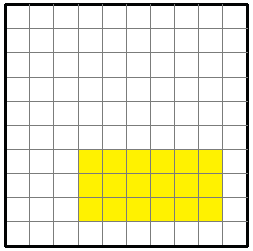### Explanation

Step 1:

Area of a rectangle = l × w; l = length = 6; w = width = 3

Step 2:

Perimeter of the rectangle = 6 + 3 + 6 + 3 = 18 units

Area of the rectangle = 6 × 3 = 18 square units.

Q 2 - Find the area and perimeter of the following rectangle.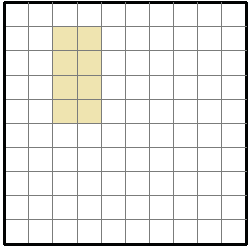### Explanation

Step 1:

Area of a rectangle = l × w; l = length = 4; w = width = 2

Step 2:

Perimeter of the rectangle = 4 + 2 + 4 + 2 = 12 units

Area of the rectangle = 4 × 2 = 8 square units.

Q 3 - Find the area and perimeter of the following rectangle.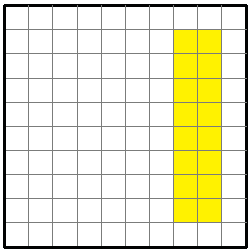### Explanation

Step 1:

Area of a rectangle = l × w; l = length = 8; w = width = 2

Step 2:

Perimeter of the rectangle = 8 + 2 + 8 + 2 = 20 units

Area of the rectangle = 8 × 2 = 16 square units.

Q 4 - Find the area and perimeter of the following rectangle.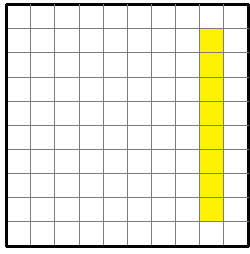### Explanation

Step 1:

Area of a rectangle = l × w; l = length = 8; w = width = 1

Step 2:

Perimeter of the rectangle = 8 + 1 + 8 + 1 = 18 units

Area of the rectangle = 8 × 1 = 8 square units.

Q 5 - Find the area and perimeter of the following rectangle.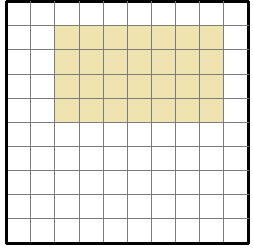### Explanation

Step 1:

Area of a rectangle = l × w; l = length = 7; w = width = 4

Step 2:

Perimeter of the rectangle = 7 + 4 + 7 + 4 = 22 units

Area of the rectangle = 7 × 4 = 28 square units.

Q 6 - Find the area and perimeter of the following rectangle.### Explanation

Step 1:

Area of a rectangle = l × w; l = length = 4; w = width = 1

Step 2:

Perimeter of the rectangle = 4 + 1 + 4 + 1 = 10 units

Area of the rectangle = 4 × 1 = 4 square units.

Q 7 - Find the area and perimeter of the following rectangle.### Explanation

Step 1:

Area of a rectangle = l × w; l = length = 6; w = width = 2

Step 2:

Perimeter of the rectangle = 6 + 2 + 6 + 2 = 16 units

Area of the rectangle = 6 × 2 = 12 square units.

Q 8 - Find the area and perimeter of the following rectangle.### Explanation

Step 1:

Area of a rectangle = l × w; l = length = 9; w = width = 2

Step 2:

Perimeter of the rectangle = 9 + 2 + 9 + 2 = 22 units

Area of the rectangle = 9 × 2 = 18 square units.

Q 9 - Find the area and perimeter of the following rectangle.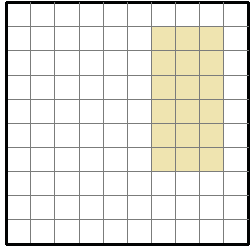### Explanation

Step 1:

Area of a rectangle = l × w; l = length = 6; w = width = 3

Step 2:

Perimeter of the rectangle = 6 + 3 + 6 + 3 = 18 units

Area of the rectangle = 6 × 3 = 18 square units.

Q 10 - Find the area and perimeter of the following rectangle.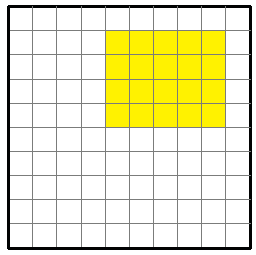### Explanation

Step 1:

Area of a rectangle = l × w; l = length = 5; w = width = 4

Step 2:

Perimeter of the rectangle = 5 + 4 + 5 + 4 = 18 units

Area of the rectangle = 5 × 4 = 20 square units.

finding_perimeter_or_area_of_rectangle_in_the_coordinate_plane.htm